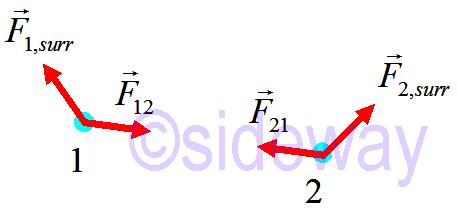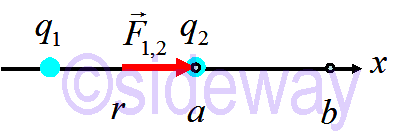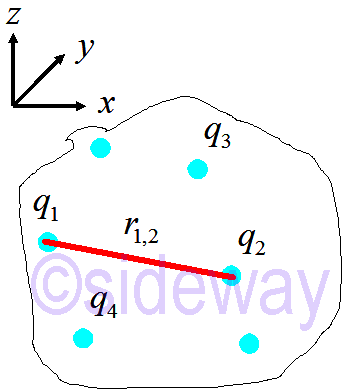output.to from Sideway
Draft for Information Only

# Content

`Energy of a Single Particle Energy Principle for a Particle Potential Energy Work Electric Potential Energy Source and Reference`

# Energy of a Single Particle

The energy of a single particle is `𝐸particle= 𝑚𝑐21−𝑣2𝑐2=𝑚𝑐2+𝐾≈𝑚𝑐2+12𝑚𝑣2=Rest energy+Kinetic Energy (𝑣≪𝑐)`

## Energy Principle for a Particle

`work done on particle=∆𝐸particle=𝑊=∫𝐹∙𝑑𝑟` Assume rest energy does not change `∆𝐸particle=𝑊=∆𝐾`

## Potential EnergyPotential energy is an interaction energy. ```∆𝐸1=𝑊on,1=∫𝐹1,surr∙𝑑𝑟+∫𝐹12∙𝑑𝑟  =𝑊1,surr+𝑊12 Work done on particle 1 ∆𝐸2=𝑊on,2=∫𝐹2,surr∙𝑑𝑟+∫𝐹21∙𝑑𝑟  =𝑊2,surr+𝑊21 work done on particle 2 ``` Consider the two particles as a system. internal work happens when the system changes shape. Potential energy is the interaction energy in a system of more than one particle. Therefore potential energy does not exist for a single particle system. ```∆(𝐸1+𝐸2)=𝑊surr+𝑊internal Total change in single particle energies ∆(𝐸1+𝐸2)=∆𝐾=𝑊surr+𝑊internal 𝑊internal+∆𝐾=𝑊surr ∆𝑈+∆𝐾=𝑊surr Multiparticle energy principle ⇒∆𝑈=−𝑊internal ```

## WorkThe work done on charge particle 𝑞2 to against the influence due to 𝑞1 is ```𝑊𝑎,𝑏=𝑏∫𝑎𝐹1,2𝑑𝑥=−𝑏∫𝑎𝑞1𝑞24𝜋𝜀0𝑟2𝑟∙𝑑𝑟=𝑞1𝑞24𝜋𝜀01𝑟𝑏｜𝑎=𝑞1𝑞24𝜋𝜀01𝑏−1𝑎 ⇒∆𝑈=𝑊surr=𝑞1𝑞24𝜋𝜀01𝑏−1𝑎≡𝑈𝑏−𝑈𝑎```

## Electric Potential Energy

According to ∆𝑈=−𝑊internal, the energy difference of a charged partcle in different position is equal to the difference of electric potential energy at different position with respect to the relative postion of the system. In other words, the electric potential energy between two charged particles can be expressed as `Electric Potential Energy 𝑈=𝑞1𝑞24𝜋𝜀0𝑟` Assume 𝑣𝑓−𝑣𝑖. Then ∆𝐾=0. Doing work on the system increases its potential energy. Work always changes 𝐸sys, so the potential energy must have changed. The electric potential energy is similar to gravitational potential energy ```𝐹=−𝐺𝑚1𝑚2𝑟2𝑟 𝑈grav=−𝐺𝑚1𝑚2𝑟 𝑈grav=−𝐺𝑚Earth𝑚𝑅Earth+ℎ For 𝑅≫ℎ; 𝑈grav≈−𝐺𝑚Earth𝑚𝑅Earth+𝑚𝑔ℎ+𝑂ℎ𝑅Earth2 ``` For multiple electric chargesThe electric potential energy of the system is `𝑈ele= ∑𝑖<𝑗𝑈𝑖𝑗= ∑𝑖<𝑗14𝜋𝜀0𝑞𝑖𝑞𝑗𝑟𝑖𝑗`

## Source and Reference

ID: 191103002 Last Updated: 30/11/2019 Revision: 0Home 5

Management

HBR 3

Information

Recreation

Culture

Chinese 1097

English 337

Computer

Hardware 151

Software

Application 198

Manim 121

Numeric 19

Programming

Web 283

Unicode 494

HTML 65

CSS 58

ASP.NET 174

OS 389

Python 19

Knowledge

Mathematics

Algebra 25

Geometry 21

Calculus 67

Engineering

Mechanical

Rigid Bodies

Statics 92

Dynamics 37

Control

Physics

Electric 27